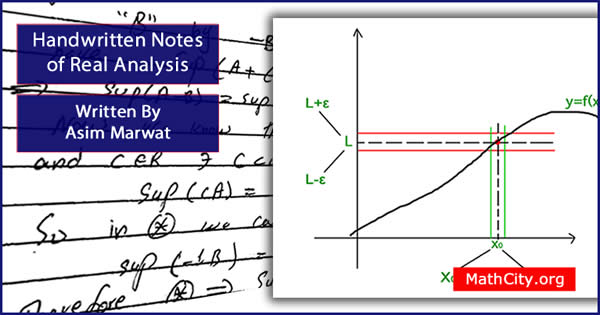# Handwritten Notes of Real Analysis by Asim MarwatThese are basic notes useful in MSc or BS mathematics for real analysis. We are really very thankful to Asim Marwat for providing these notes and appreciates his effort to publish these notes on MathCity.org

 Name Handwritten Notes of Real Analysis Asim Marwat PDF (see Software section for PDF Reader) Different chapter have different size
• Chapter 01: Real Numbers
• Bounded above set, least upper bound (lub), bounded below set, greatest lower bound (glb), Archimedean property, density theorem, Bolzano Weierstrass theorem.
• Chapter 02: Limit of the Function
• Sequence, bounded sequence, Sandwhich theorem, monotone sequence, monotonic convergent theorem, nested intervals, sub-sequence, Cauchy sequence, comparison test, limit comparison test, D. Alembert test (ratio test), integral test, absolute convergent, root test.
• Chapter 03: Limit of a Function
• Right hand limit, signum function, intermediate value theorem, fixed point, uniform continuity.
• Chapter 04: Derivative
• Rolle's theorem, Lagrange's mean value theorem, Cauchy mean value theorem, monotone functions, critical point.
• Chapter 05: Riemann Theory of Integration
• Partition of domain of the function, upper sum, lower sum, refinement of a partition, Riemann integration, fundamental theorem of calculus,
• Chapter 06: Function of Several Variables
• Jacobian, extreme values, extreme value of a function of several variable.
• msc/notes/handwritten-notes-real-analysis-asim_marwat